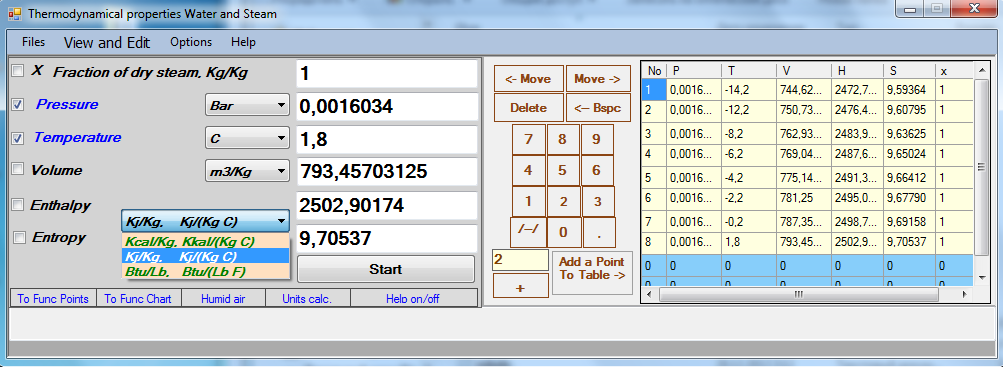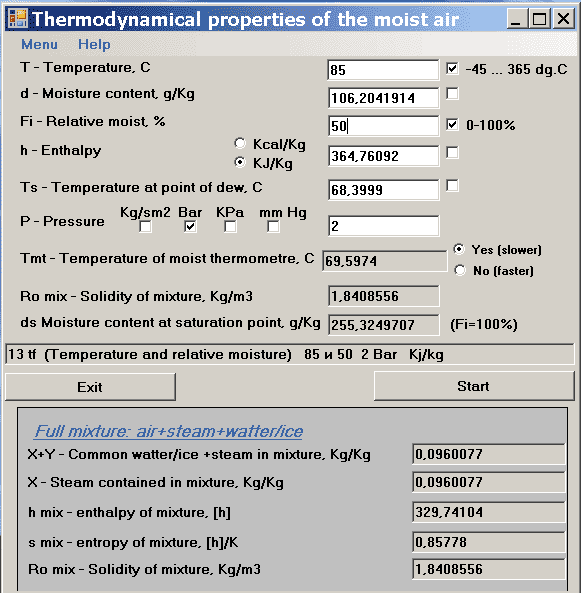Steam And Water rus
 SteamAndWater Calculator - the tool for calculating of termodynamic properties of water, water steam and humid air.
The program contains two important windows: - a window of thermodynamic properties of Steam and Water, and a window of thermodynamic properties of the Humid Air.The window of thermodynamic properties Steam and Water calculates p,t,v,h,s,x having 2 of p,t,v,h,s: (p,t); (p,v); (p,h); (p,s); (t,v); as input parameters, excluding saturation curve, and two-faze zone where only (p,x); (t,x); (v,x); (s,x) pairs can be used as input.

The pair of (p,t) has the most wide limits:
P=0,0000408...300 ata(Kg/sm2, absolute) for T=0...550 C
and
P=0,0000408...0,99999 ŕňŕ for T=-50...0 C.

The pairs of (p,h) and (p,s) have limits:
p=0,0000408...300 ata, and limits of s or h depend on specified pressure.

Following units can be selected as an input/output units:
p - Kg/sm2, Bar, kPa, Psi;
t - C, K, F;
v - m3/kg, Ft3/Lb;
h - Kj/Kg, Kcal/Kg, Btu/(Lb);
s - Kj/(Kg K), Kcal/(Kg K), Btu/(Lb F).

The next important window is the window of calculator of the termodynamic properties of the humid air.

Following pairs are accessible as input parametres:
(T,d); (T,Fi); (T,h); (T,Ts);
(d,Fi); (d,h);
(Fi,h); (Fi,Ts);
(h,Ts).

The temperature T - must belong to an interval -45...365 Ń.
The pressure P for each a pair must belong to an interval 0,0000408 Kg/sm2 ... 225Kg/sm2.This demo version is unlimited by the use time and has a good functionality: